Charge Radii of Mirror Nuclei and the Neutron Matter Equation of State

(2017)
The difference in the root-mean-square charge radii of mirror nuclei is shown to be a robust measure of the derivative of the symmetry energy and the neutron equation of state near nuclear saturation density. Due to Coulomb distortion, the neutron skin contains a extra term proportional to the value of the symmetry energy.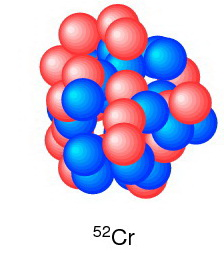The Music of Nuclear Energy Level Spacings

(2017)
The spacings for the complete set of energy levels up to 6.2 MeV in 208Pb have been analyzed in terms of GOE and Poisson distributions. The experimental result is in close agreement with the GOE  distribution. It is also in agreement with calculations based on a realistic residual interaction. The energy spacing have been converted into musical notes so that one can hear as well as see the difference.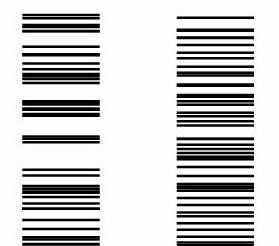A Nuclear Detective Story

(2015)
The 30P(p,gamma)31S rapid-proton capture rate is one of the most important reactions that determines the fractions of elements up to calcium that are produced in novae. We are trying to match up the experimental and theoretical level-scheme finger prints for  31S in order to reduce the uncertainty in this reaction rate.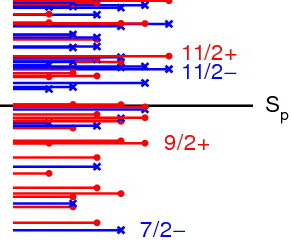Collaboration Between Experiment and Theory

(2015)
The theory group at the NSCL has expertise in many theoretical methods. Collaboration among the members of group and with the experimental program  at the NSCL provide the resources and interactions needed to make advances toward the overall goals of nuclear physics.### The Puzzle of 46Ar

(2015)
New mass measurements of 48Ar and 49Ar obtained at the NSCL are in excellent agreement with theory. Combined with AME 2012 masses they show, for first time, evidence for a shell gap at N=28 for argon. The experimental B(E2) at N=28 in 46Ar shows a reduction as qualitatively expected, but in contradiction to theory.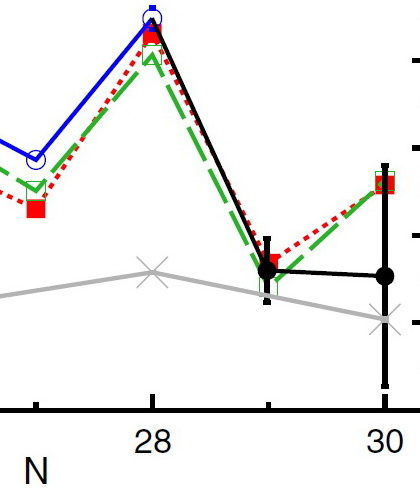### Neutrinoless Double Beta Decay

(2014)
A new method is developed for calculating the nuclear matrix elements for neutrinoless double beta decay in nuclei in terms of an expansion over intermediate states of the nucleus with two less nucleons. We show that the matrix element is dominated by a transition through the ground state of this nucleus.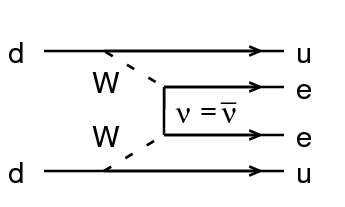### Modification of Brink-Axel

(2014)
The shell model is used to calculate magnetic dipole (M1) and Gamow-Teller transitions from states at high excitation energy. The calculations for M1 explain the low energy enhancement observed in the gamma decay spectrum of Fe. Based on these results we propose a modification to the Bink-Axel hypothesis.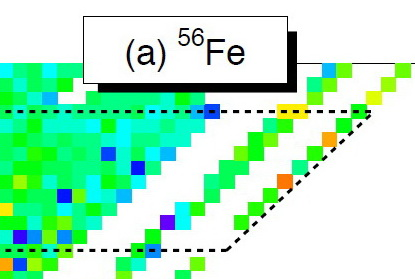### Level Density

(2014)
New statistical methods have been developed for the calculation of level densities in the shell model basis. In cases where the they can be compared to the exact results the agreement is excellent.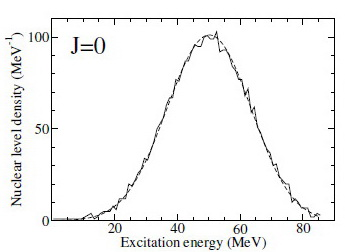### The Neutron Equation of State

(2014)
For the first time we have used ab initio calculations for low-density neutron matter together with data for the magic nuclei to constrain the Skryme form for the neutron equation of state for densities up to about 0.20 nucleons per fermi cubed.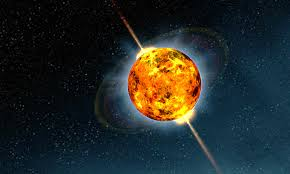### NuShellX@MSU

(2014)
NuShellX@MSU is a powerful and widely used suite of computer programs for nuclear Configuration Interaction calculations of wavefunctions, energies and overlap matrix elements.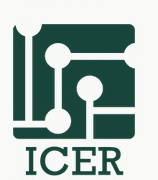### Boundary Between CI and EDF

(2011)
A new method is proposed for combining features of configuration interaction theory and energy density functional theory.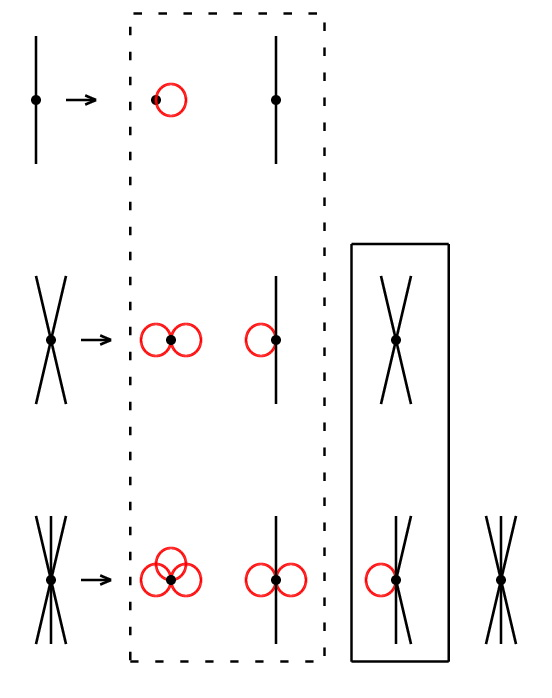### Fighting for Subatomic Independence

(2011)
The correlations of deeply bound protons with loosely bound neutrons is revealed to reduce the nucleon removal overlap.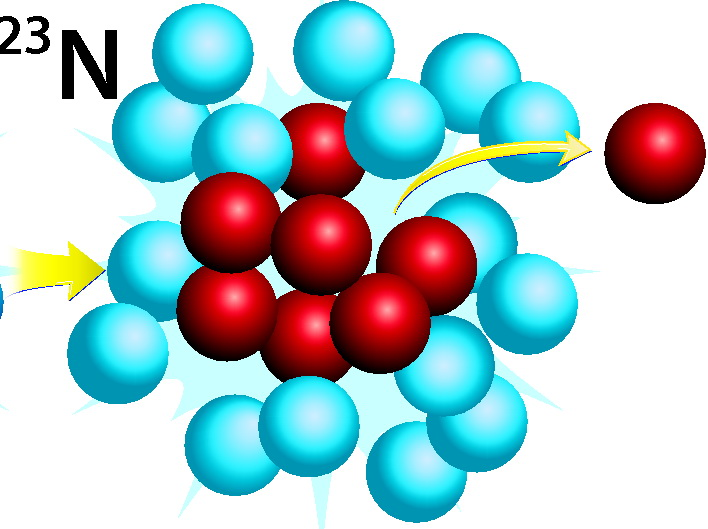### Chaos and the Continuum

(2011)
The unexpected results for the deviation of resonance widths from the expeted Porter-Thomas shape are explained by theory which contains coupling to the continuum.  The phenomenon is of general nature and it may influence all processes of signal transmission through a quantum system.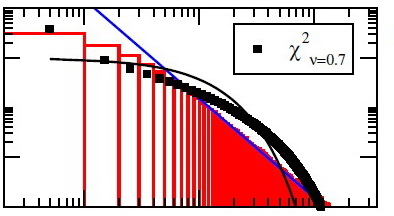### Search for the Three-Phonon Quadrupole

(2011)
Theoretical predictions for gamma decay E2 strengths  in 62Ni are compared to new experimentsl results in the serach for evidence for decay of a possible three-phonon multiplet.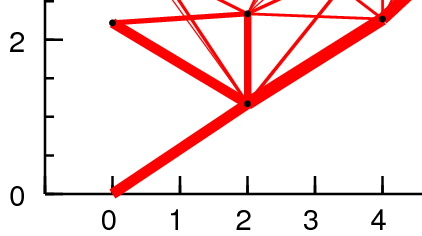### Beyond the Neutron Drip Line

(2010)
Predictions for properties nuetron unbound states in the nuetron-rich isotopes of oxygen are compared to recent experiments.### Islands of Shell Breaking

(2010)
Regions of nuclei near the neutron drip line show that the traditional shell model magic numbers are often changed. The physics behind these changes is discussed here.